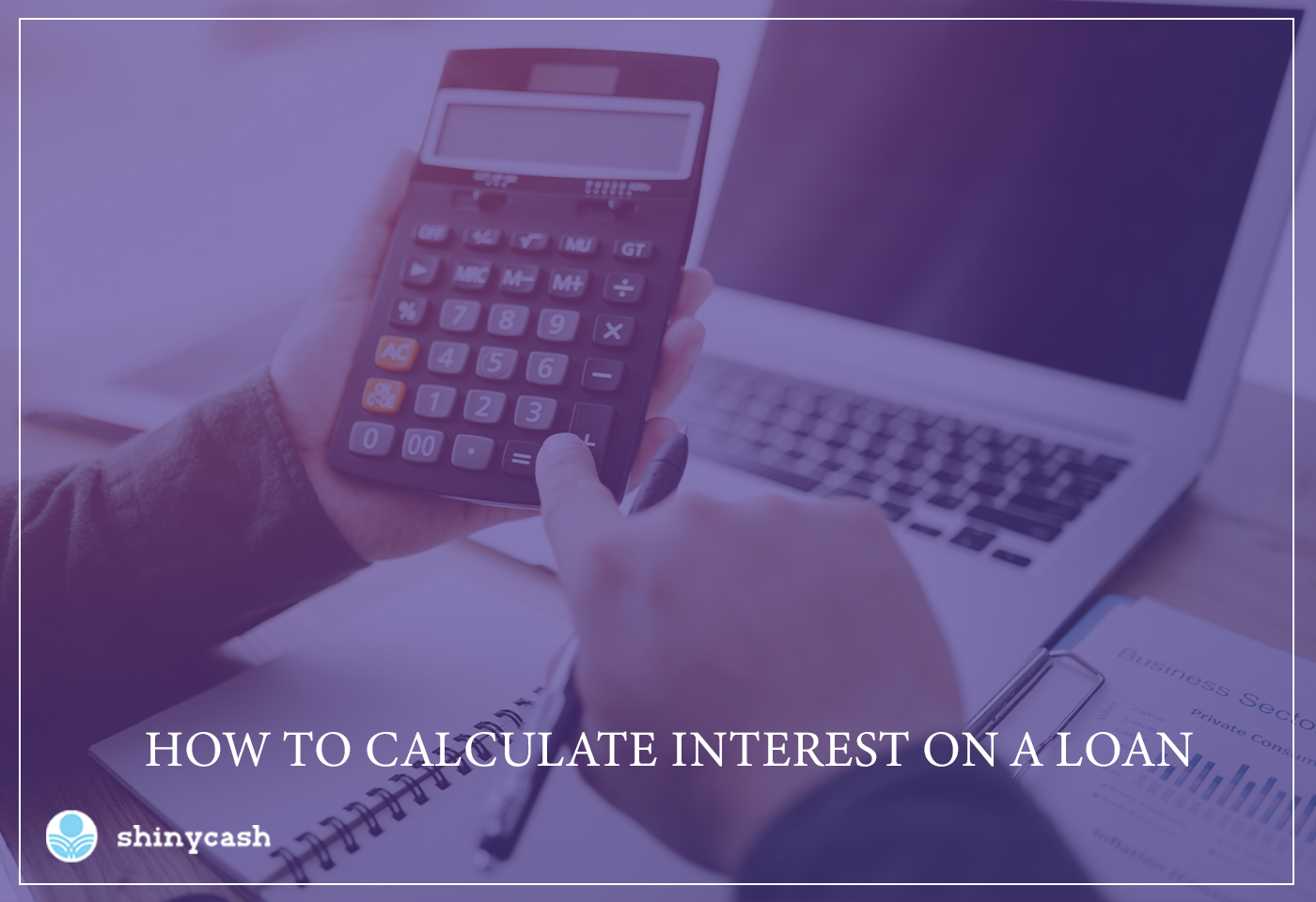# How to Calculate Interest on a Loan?

October 15, 2021

Being in debt is a pain. Indeed, nothing is more awkward than having to pay all those interest charges each month when you might be spending your money on something else. Today, we will explain how interest on your loan is calculated to help you understand how much your debt costs you each month.

## Interest Rate Calculation Explained

Calculating interest on a loan is a pretty straightforward and direct deal. First, you need to have the interest rate on the loan you consider and the principal amount you owe. For example, assume you have a personal loan with an 8% annual interest rate and a total loan balance of \$15,000.

First Step: First things first, begin by dividing the interest rate by one hundred to convert the percentage to a decimal. In this scenario, you get 0,08.

Second Step: Next, you need to multiply that number by the principal amount of the loan and get \$1,200. This figure shows the interest rate that would pile up on your loan annually.

Third Step: To calculate the average monthly interest rate, you need to divide that \$1,200 by twelve, which results in \$100 per month. This would be the exact amount you should expect to see in your monthly statement. Take into consideration that the interest rate may change from month to month, based on the number of days in a particular month or when exactly your payment is processed.

## Example of an Auto Loan

People now frequently go for loans of fifty, sixty, or even sometimes a hundred thousand dollars for items like a new automobile. Let's say you opt for an auto loan of \$100,000 at an interest rate of 10%. The interest alone would be \$10,000 a year and more than \$800 per month. That's just the interest that banks or other lending institutions make on these loans. With that sort of benefit, it's not surprising why they're so eager to give you a new auto loan with no down payment!

### Extra Payment Means Less Interest

This formula of credit repayment also works in reverse; that's why many borrowers decide on making additional monthly installments on loans. For example, assume you have \$500 set aside in your budget to pay off one of your outstanding debts. You may use this formula to determine how much less interest you'll have to pay on that loan in the future. As before, let's begin by dividing the interest rate of 10% by one hundred to get a decimal number of 0,10. Next, multiply that number by the additional \$500 and end up with \$50 in your pocket. That's how much interest you'd have had to pay over the following twelve months if you hadn't made this extra payment. That's a total of \$50 that you can now retain for yourself.

### The Bottom Line

We strongly encourage you to practice these calculations with some of your loans, credit cards, or payday loans to understand how much your debt is costing you. Figuring this out may truly encourage you to do everything possible to pay off your debts quickly.